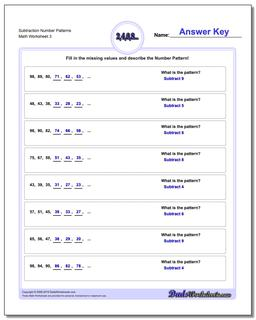# Math Worksheets: Number Patterns: Number Patterns: Subtraction Number Patterns (Third Worksheet)## Subtraction Number Patterns (Third Worksheet)

PropertyValue
DescriptionSubtraction Number Patterns: Number pattern problems using only subtraction operations. (Third Worksheet)
Resource TypeWorksheet